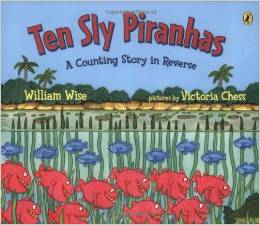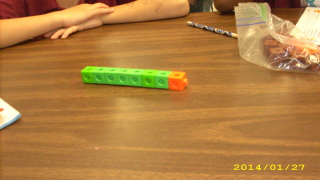# Use Addition to Check Subtraction

18 teachers like this lesson
Print Lesson

## Objective

SWBAT apply the inverse relationship of addition and subtraction.

#### Big Idea

Undo what can be undone! In this lesson, students will use addition to check subtraction to make sure their answer is correct.

## Activating Strategy

10 minutes

I begin this lesson by reading the story "Ten Sly Piranhas"If the book isn't available, there is a video available:

I then show a cube train of 10 cubes.  I ask the students “How many cubes are there?”  I then break off some cubes and hide them.  To guide their thinking on how addition and subtraction are related, I ask them:

• How many cubes do you see now? (I see six cubes)
• How many are hidden?  (4 cubes are hidden)
• How do you know? (you started with 10 cubes, and you now have 6.  I know that 6 + 4 = 10; or I know that 10 – 6 = 4)

I would then repeat the activity with another cube train and ask students to write the subtraction sentences.

Understanding why addition and subtraction are inverse relationships is the key to working with various types of problems and developing fluency in computation.

• The inverse relationship between addition and subtraction can make memorizing number facts easier for children.

• Children should understand that one operation undoes the other and that they can use addition to check their subtraction.

• Understanding these relationships is important for later work in algebra. For example, if 14 – 8 = 6, then 6 + 8 =14.

## Teaching Strategies

15 minutes

I put the following word problem on the board or on chart paper: (available as a PPT Use addition to check subtraction)

Jane has 11 books.  She gives 4 of them to Pat.  How many books does Jane still have?

I guide the discussion:

• Do we need to add or subtract? (subtract)
• Why? (I need to find out how many books Erin still has)

After giving children time to solve the above problem, I would then ask them to write a related addition sentence.

Since the key idea of this lesson is to get the students to relate the inverse relationship of addition and subtraction, I then write another example of a related addition and subtraction sentence on the board/chart paper.

• Example: 15 – 8 = 7       7 + 8 = 15

• How can I use addition to check my subtraction? (since I know that related facts have the same numbers in them, I can use addition to check my subtraction)

To further extend the guided practice portion of the lesson, I write more examples on the board/chart paper. (also found on the Use addition to check subtraction)

In this picture, a student is making a model using connecting cubes to work on a math problem:## Independent Practice

30 minutes

If the students correctly answered the guided practice questions, I then assign Use addition to check subtraction worksheet.  These are similar problems to the guided practice problems.  To extend their thinking, I will write a couple of subtraction problems on the board, and ask them to use addition to check the answers.  I have them explain how to correct the incorrect sentence.

• 11 – 5 = 6
• 12 – 5 = 8

If a student is struggling with the concept while in a small group, I have them make a model of a subtraction sentence.  By having them create a concrete model, it will help them move from the concrete to the abstract concept of the relationship between addition and subtraction.  (MP5)

Give students 10 connecting cubes.  Have them model the following subtraction sentence:

• 9 – 2 = ___

I guide the discussion using the following questioning techniques:

• How many cubes are in the cube train?  ( 9 )
• How many cubes do you need to subtract to find the difference? ( 2 cubes)
• Have students remove 2 cubes from the cube train
• How many are left in the cube train? (7 cubes)
• To check you work, add 2 and 7 to see if you get 9

In this video, a student is using counters to model her subtraction problem.

## Closing/Summarizing

5 minutes

To close out the lesson, I call the students back to the carpet and have them share with a shoulder partner how we can use addition to check subtraction.